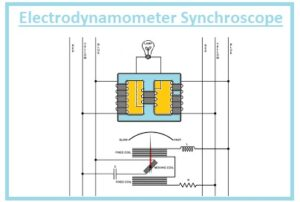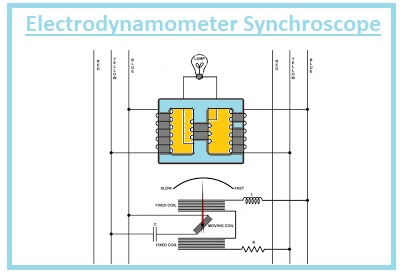Hello, readers welcome to new post. Here we will discuss What is Synchroscope. There is different instruments used to find the value of angle among the ac systems such as generators that has synchronization. Such a system is called synchronize that has the same value of frequency and phase angle 0. To measure the angle between synchronized devices there is synchroscope was used.

If the measured angle is zero these systems will make connections to each otherwise no. Here we will learn the details about the synchroscope and its other parameters. So let gets started

## What is Synchroscope

• The device is used to find the angle among the two AC systems such as the generator connected and has synchronization called synchroscope.
• The term synchronized explain the frequency of two systems having similar value with zero phase angle.
• After finding the value of phase angle and frequency of system values shown on the meter.
• If these terms have 0 values then link the two devices to the system.
• If there is no same frequency and phase angle zero larger amount of current will flow  and harm any devices working as protection such as breakers and fuses
• There is 2 phase that exists at wound shape stator and rotor. It works on the 2 phases power supply.
• If there is synchronization occurs among 2 phases then for third will come automatically.### Working Principle of Synchnoscope

• The working operation of this device is like the AC motors. Its rotation starts when there is a difference exist among the running and incoming generator frequencies.
• The needle of the synchroscope will show rotation in clockwise if there is a large value of frequency for the incoming generator.
• During its operation, it gets the 2 phase power from the alternator,

### Synchroscope Construction

• In the construction of this device, there are 2 poles that lie at the incoming generator that linked about the 2 phases.
• At armatures, there is 2 parallel combination linked to the same phase in switch buses.
• There is resistance linked at half part of one winding and inductance in series combination to another half of windings.
• The inductance moves the current at ninety degrees according to the current in resistance.
• These 2 current moves to armature windings to generate a rotating field along the poles.

### Types of Synchroscope

• There are 2 types of synchroscope
• Electrodynamometer Synchroscope
• Moving Iron Synchroscope

## Electrodynamometer Synchroscope

• It comprises of stationary and moveable portions that are the main components of this device.
• There is three limb transformer is linked at the stationary portion and lamp.
• There is a supply for excitation of one winding of the transformer is get through bus bar and other two get excitation from machines.
• The connection of the lamp is made to the mid limb of the transformer.
• Flux is generated through the extreme limb of the transformer and flux at mid limb is generated through a combination of other two.
• The external limb of the transformer is linked in such a technique that if the incoming devices are synchronized then extreme voltages are generated in the mid limb of the transformer and the lamp glows out..
• If volts of incoming devices is not synchronized then there resultant flux for the mid limb of the transformer is 0 and the lamp woll, not show light.
• Due to not same values of frequency of incoming machines and bus bar lamp gets flicker.### Moving Iron Synchroscope

• There is 2 immoveable portion it has. One portion is operating form a small level current and resistance is linked series combination to it.
• It has two cylinders that are placed at the spindle. Pressure winding is used to excitation these cylinders.
• Pressure windings is attached to 2 phase of incoming devices. The resistance is linked in a series combination to one pressure windings and inductance to other windings.• Due to inductance and resistance, there is a phase difference of ninety degrees exist among the pressure windings.
• If the frequency of incoming devices and bus bar is the same spindle moves, Rotation during one second defines teh differences among the values of frequency.

That is all about the synchroscope. I have explained each and every parameter related to this device with detail. If you have any further query ask in the comments. Thanks for reading have a good day see you in next post.